# Excel DROP function to remove rows or columns from range or array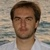by , updated on

This tutorial introduces you to the brand-new Excel 365 function that can drop unwanted rows and columns to return a relevant subset of data.

When analyzing huge datasets, you may sometimes need to pull only the data without a total row/column, headers or footers. In earlier Excel versions, you'd have to rely on the good old copy/pasting technique. In Excel 365, we have a special function to dynamically extract the desired part of the array by "dropping" irrelevant data.

## Excel DROP function

The DROP function in Excel removes the specified number of rows and/or columns from the start or end of an array.

The syntax includes the following arguments:

DROP(array, rows, [columns])

Where:

Array (required) - the source array or range.

Rows (optional) - the number of rows to drop. A positive value removes rows from the start of the array and a negative value from the end of the array. If omitted, columns must be set.

Columns (optional) - the number of columns to drop. A positive integer excludes columns from the start of the array and a negative integer from the end of the array. If omitted, rows must be defined.

Here's the DROP function in action: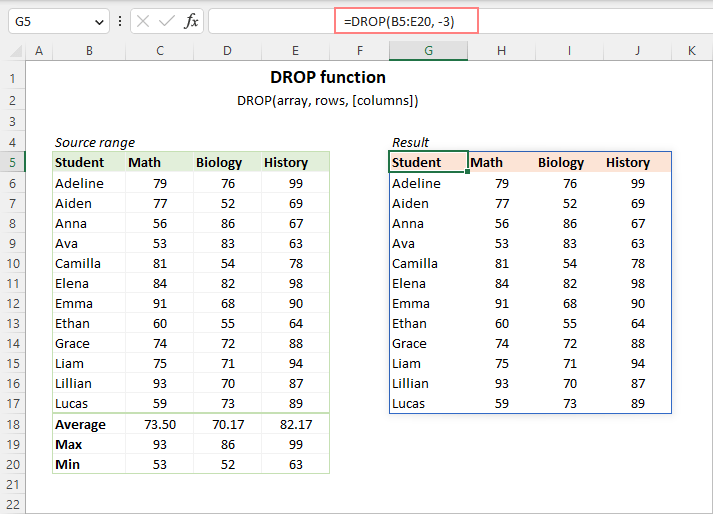### DROP function availability

The DROP function is only available in Excel for Microsoft 365 (for Windows and Mac) and Excel for the web.

### How DROP function works

The 5 simple facts below will help you better understand the inner mechanics of the DROP function:

1. DROP is a dynamic array function. You enter the formula in the upper left cell of the destination range, and it automatically spills the results into as many columns and rows as needed.
2. The array argument can be a range of cells, an array constant, or an array of values returned by another function.
3. The rows and columns arguments can be positive or negative integers. Positive numbers drop rows/columns from the start of the array; negative numbers - from the end.
4. The rows and columns arguments are optional, but at least one of them should be set in a formula. The omitted argument defaults to zero.
5. If the absolute value of rows or columns is greater than or equal to the total number of rows and columns in the array, a #CALC! error is returned.

## How to use DROP function in Excel - formula examples

Armed with theory, let's move on to practical matters and see how you can return part of an array by dropping unnecessary rows or columns.

### Drop rows from start of an array

To remove a certain number of rows from the start of a 2D array or range, supply a positive number for the rows argument.

For example, to drop the first 5 rows from the range A3:C16, the formula is:

`=DROP(A3:C16, 5)`

The formula is entered in cell E3 and automatically populates the results into as many rows and columns as necessary.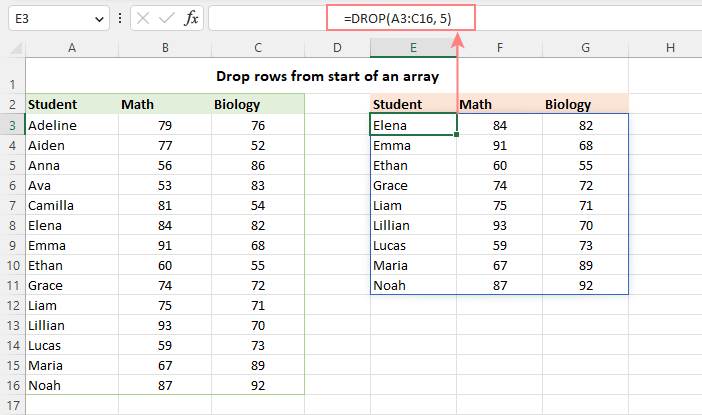### Drop columns from start of an array

To remove a given number of columns from the beginning of a 2D array or range, supply a positive number for the columns argument.

For example, to drop the first column from the range A3:C16, use this formula:

`=DROP(A3:C16, ,1)`

The formula lands in cell E3 and spills into two columns and as many rows as there are in the original range.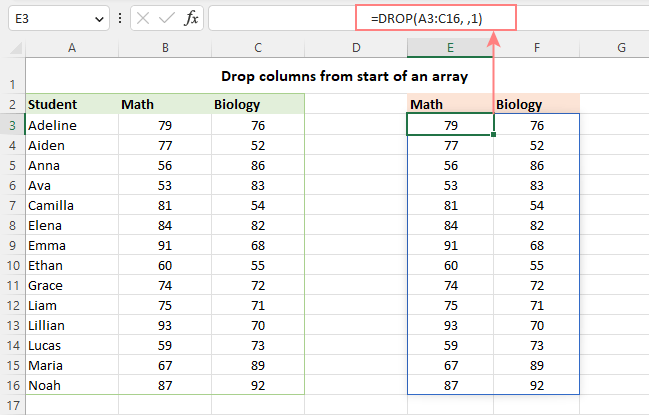### Drop rows and columns at a time

To remove both rows and columns from the beginning of an array, provide positive numbers for both the rows and columns arguments.

For example, to take away the first 5 rows and 1 column from our dataset, the formula is:

`=DROP(A3:C16, 5, 1)`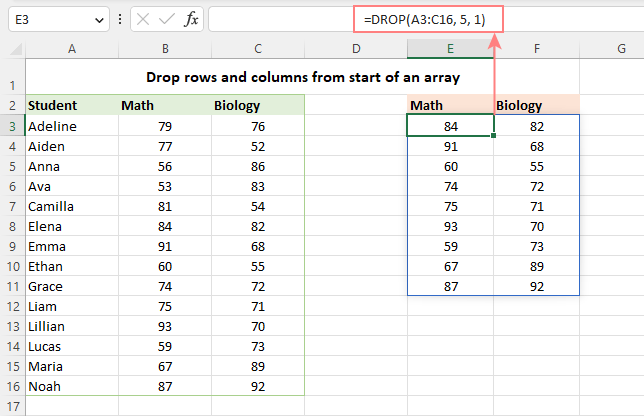### Drop last N rows

To remove rows from the end of an array, use a negative number for the rows argument. For example:

To remove the last row, supply -1:

`=DROP(A3:C16, -1)`

To exclude the last 3 rows, supply -3:

`=DROP(A3:C16, -3)`

To remove the last 5 rows, supply -5:

`=DROP(A3:C16, -5)`

The screenshot below shows the result of the last formula.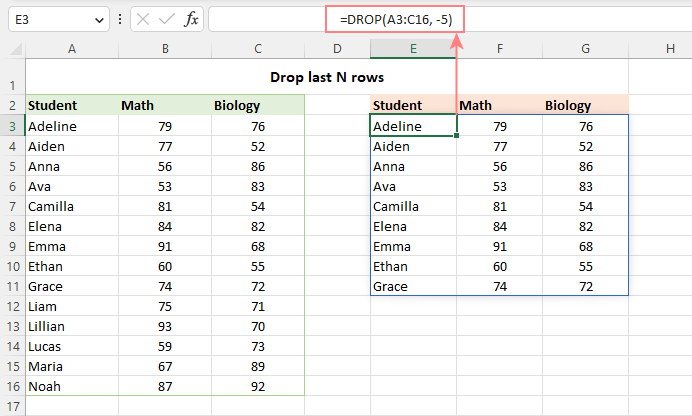### Drop last N columns

To exclude a few columns from the end of an array, provide a negative number for the columns argument. For example:

To remove the last column, use -1:

`=DROP(A3:D16, , -1)`

To drop the last 2 columns, use -2:

`=DROP(A3:D16, , -2)`

To remove the last 3 columns, use -3:

`=DROP(A3:D16, , -3)`

And here is the result with the last two columns dropped: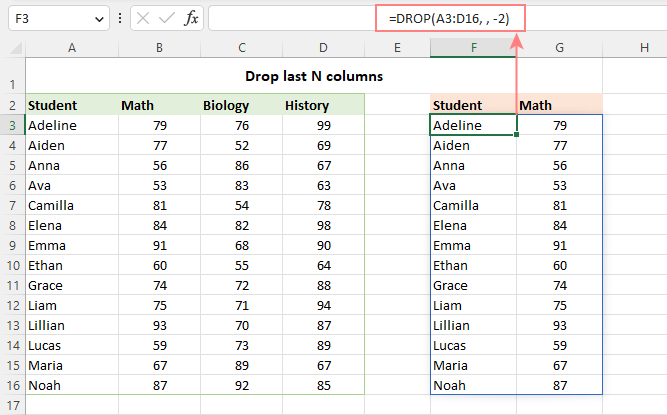Tip. To remove both rows and columns from the end of an array, provide negative numbers for both the rows and columns arguments.

## Sort and drop

In situation when you want to re-arrange the values in the source array before dropping some columns or rows, leverage the SORT function that can sort the array by any column you want in ascending or descending order.

For example, you can sort the below range by the 4th column (named Average) from highest to lowest using this formula:

`=SORT(A3:D16, 4, -1),`

And then serve the sorted array to the DROP function instructing it to omit the 5 lowest results:

`=DROP(SORT(A3:D16, 4, -1), -5)`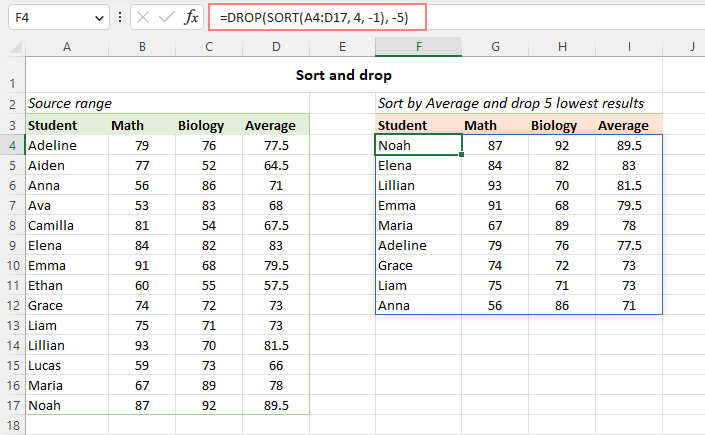## How to drop rows / columns from multiple ranges

When working with several non-contiguous ranges, you can "merge and drop" at once by combining a couple of functions in one formula:

1. Stack multiple ranges into one using the VSTACK or HSTACK function.
2. Remove a certain number of rows or columns from the stacked array using the DROP function.

Depending on how your original data is structured, one of the following approaches may work for you.

### Combine ranges vertically and drop rows or columns

Suppose you have 3 separate ranges like shown in the screenshot below. To merge the ranges vertically from top to bottom, the VSTACK formula is:

`=VSTACK(A4:D7, A11:D15, A19:D23)`

Nest it in the array argument of DROP, specify how many rows or columns to remove, and you will get the result you are looking for.

For instance, to remove the last column from the stacked array, the formula takes this form:

`=DROP(VSTACK(A4:D7, A11:D15, A19:D23), , -1)`

And the result looks as follows: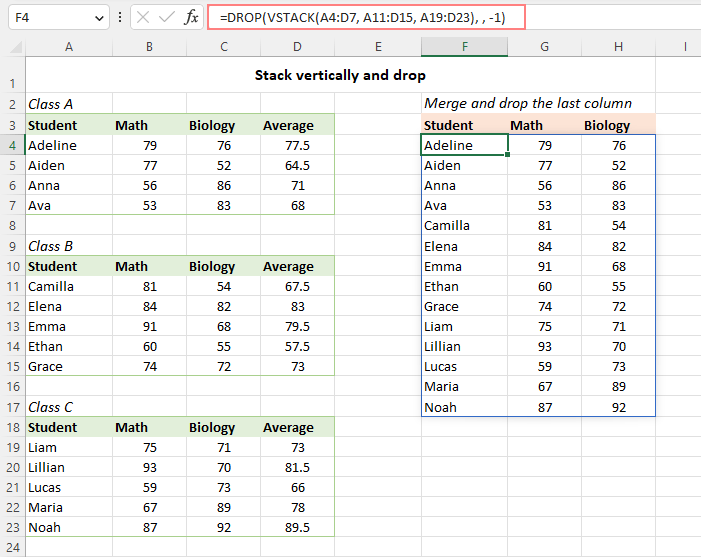### Combine ranges horizontally and drop rows or columns

In case the source ranges are arranged horizontally in rows, you can combine them into a single array with the help of the HSTACK function. In our case, this is the formula to use:

`=HSTACK(B3:E6, H3:K6)`

And then, you nest the above formula in the array argument of the DROP function and configure the rows and columns arguments as needed.

In this example, we use -1 for the row argument to remove the last row:

`=DROP(HSTACK(B3:E6, H3:K6), -1)`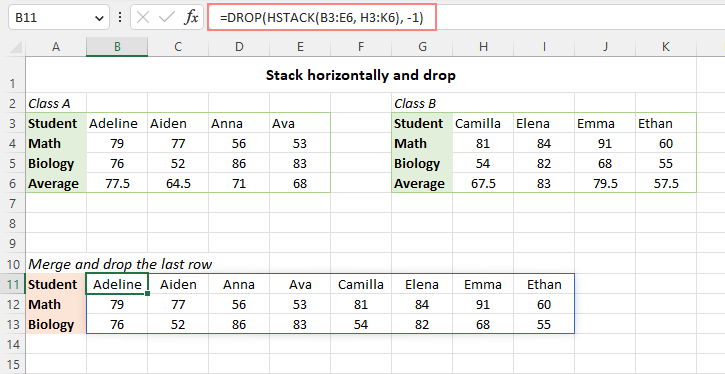## DROP vs. TAKE in Excel

Excel 365 has one more useful function to return part of an array or range - TAKE function. Essentially, these two functions perform the same task but in different ways. While DROP excludes certain rows and/or columns from an array, TAKE gets specific rows and/or columns from an array.

For example:

To remove the first 3 rows, you use:

`=DROP(array, 3)`

To extract the first 3 rows, you use:

`=TAKE(array, 3)`

## Excel DROP function not working

In case the DROP function does not work in your Excel or results in an error, it's most likely to be one of these reasons.

### DROP is not available in your version of Excel

DROP is a new function with limited availability. If your version is other than Excel 365, the formula will return a #NAME error as if you misspelled the function's name.

### Empty array

If your DROP formula is configured to remove all or more rows or columns than there are in the array, a #CALC! error will indicate that there is nothing to return.

### Not enough blank cells to output the results

In case there are not enough empty cells below or/and to the right of the formula, a #SPILL error occurs. To fix it, just clear the spill range. For more details, see How to fix #SPILL! error in Excel.

That's how to use the DROP function in Excel to remove rows or columns from an array. I thank you for reading and hope to see you on our blog next week!